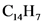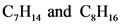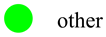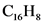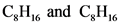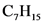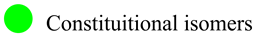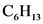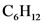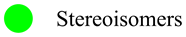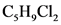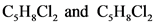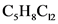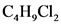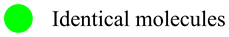# Identify each pair of compounds as constitutional isomers

1. Identify each pair of compounds as constitutional isomers,
stereoisomers, identical molecules, or other.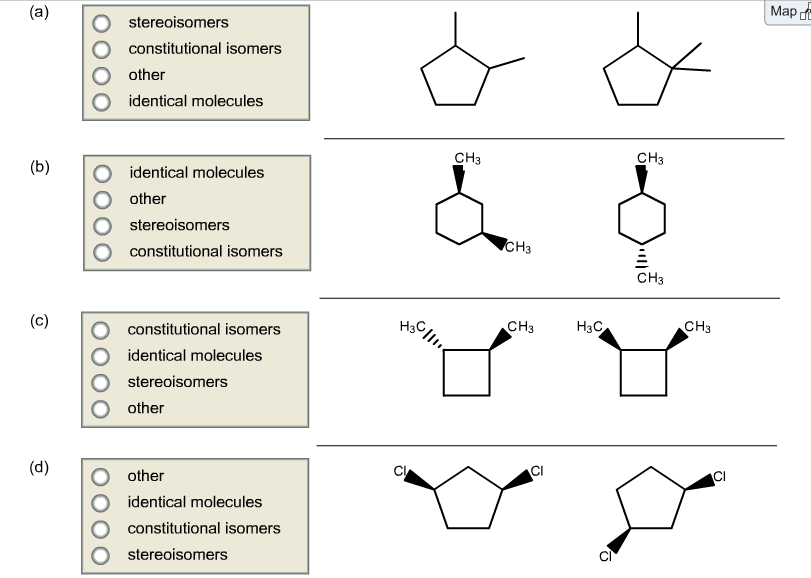(a) stereoisomers constitutional isomers other identical molecules (b)identical molecules other stereoisomers constitutional isomers (c) constitutional isomers identical molecules stereoisomers other (d)other identical molecules constitutional isomers stereoisomers

General guidance

Concepts and reason
Structural relationship between the molecules can be identified based on the arrangement of atoms in the given molecules. This relationship can be of different types namely constitutional isomers and stereoisomers. Pairs of compounds are given; relationship between each pair can be identified using the spatial arrangements of atom.

Fundamentals

Isomers are pairs of compounds, which have same empirical formula but differ in their atomic arrangement or its spatial orientation.
1. Constitutional isomers differ in connectivity of atoms in the molecule.
2. Stereoisomers differ in the spatial orientation of atoms in the molecule.
Stereoisomers are of two types namely enantiomers and diastereomers. Enantiomers are pair of compounds, which are non-superimposable mirror images of each other. Diastereomers are the pair of compounds, which does not have mirror image.
3. Identical molecules are the pair of molecules, which does not have any special relationship with each other.
Block diagram of the above explanation is given below:

Step-by-step

Step 1 of 16

Part 1.a
Structural formulas of two compounds are

Chemical formula has found by calculating the number of Carbons and Hydrogens present in the structural formula.

Take care while counting the number of constituents present in the molecule; do not inter change number between the different constituents.
Ex: molecular formula is wrong way of representation.

Compare the two chemical formulas to give relation between them.

Step 2 of 16

Relationship between two chemical formulae:
: Not isomers

Part 1.a

The chemical formula of the given pair of compounds is different. The first compound has chemical formula of C7H14while the second compound has a chemical formula of C8H16 that shows the pair of compounds having different chemical formula. Therefore, they are not isomers; they belong to a) other category.

Take care while writing the chemical formula of the compounds. Indicate the correct number of carbons and hydrogens present.
Ex: is not correct way of representation

Draw the structural formula to give the chemical formula of the compound.

Step 3 of 16

Part 1.b
Structural formulas of two compounds are

The chemical formula has written by calculating the number of Carbons and Hydrogens present in the structural formula.

Take care while counting the number of constituents present in the molecule; do not inter change number between the different constituents.
Example: the molecular formula is the wrong way of representation.

Also Read :   Why did the Grizzly go on a diet?

Compare the two chemical formulas to give there lation between them.

Step 4 of 16

The relationship between two chemical formulae:
: Isomers

The chemical formula of both the compounds are same, C8H16. A pair of compounds having a same chemical formula is known as isomers. Hence, the given pairs are isomers.

Take care while writing the chemical formula of the compounds. Indicate the correct number of carbons and hydrogen present.
Example:is the wrong way of representation.

Look for the positions of substituents connected.

Step 5 of 16

The position of substituents in the given pairs of compounds is given below:

The connectivity of substituents is written by determining the position of substituents attached to the cyclohexane ring.

Take care while giving the substitution; do not interchange the positions of the molecules.
Example: instead of 1, 3-substitution do not give 1, 4-substitution

Compare the relationship between the pairs using the position of substituents.

Step 6 of 16

The relationship between the pairs using the position of substituents is:
1, 3-substitution and 1, 4-substitution: Constitutional isomers

Part 1.b

The connectivity of both the compounds is not same. In the first compound, the two methyl groups are substituted at 1, 3-position. But in the second compound, the two methyl groups are substituted at 1, 4-position. A pair of compounds with same chemical formula and different connectivity of atoms is known as constitutional isomers. Hence, they are constitutional isomers.

Take care while indicating the position of substituents present in the given molecule; do not give wrong substitution position.
Example: instead of 1, 3-substitution do not give 1, 5-substitution

Draw the structural formula to give the chemical formula of the compound.

Step 7 of 16

Part 1.c
Draw the chemical structures given of each compound in part c

The chemical formula has written by calculating the number of Carbons and Hydrogens present in the structural formula of the compounds.

Take care while counting a number of hydrogen; do not give excess number.
Example:

Compare the relationship between two chemical formulae.

Step 8 of 16

The relationship between two chemical formulae:
: Isomers

The chemical formula of both the compounds is same,.Pair of compounds having same chemical formula are known as isomers. Hence, they are isomers.

Take care while writing the chemical formula of the compounds. Indicate the correct number of carbons and hydrogens present.

Look for the position of substituents connectivity.

Step 9 of 16

The position of substituents in the given pairs of compounds is given below:

Also Read :   5 Tips for Success as a Fashion Amazon Business

The connectivity of substituents is written by determining the position of substituents attached to the cyclobutane ring.

Take care while giving the substitution; do not interchange the positions of the molecules.
Example: instead of 1, 2-substitution do not give 1, 4-substitution

Compare the relationship between the pairs using theposition of substituents.

Step 10 of 16

The relationship between the pairs using theposition of substituents is:
1, 2-substitution and 1, 2-substitution: Stereoisomers

The connectivity of both the compounds is same. In both cases, the two methyl groups are substituted at 1, 2-positions of the cyclobutane ring. Hence they are stereoisomers.

Take care to indicate the position of substituents present in the given molecule.
Ex: instead of 1, 2-substitution do not give 1, 4-substitution

Look for the spatial arrangement of the substituents.

Step 11 of 16

The spatial arrangements of atoms are:

Part 1.c

The stereochemistry or the special orientation of the substituents is different. The first compound has (S, S) stereochemistry and the second compound has (R,S) stereochemistry. A pair of compounds which have same chemical formula and same connectivity but differ only in their spatial orientation is known as stereoisomers. Hence, they are stereoisomers.

Take care while indicating the correct stereochemistry of the atoms.
Example: do not give S-configuration instead of R-configuration.

Draw the structural formula to give the chemical formula of the compound.

Step 12 of 16

Part 1.d
Chemical structures the given compounds.

The chemical formula has written by calculating the number of Carbons and Hydrogens present in the structural formula.

Take care while counting a number of hydrogen; do not give excess number.
Example:

Compare the two chemical formulas to give therelation between them.

Step 13 of 16

The relationship between two chemical formulae:
: Isomers

The chemical formula of both the compounds is same,. A pair of compounds having same chemical formula is known as isomers. Hence, they are isomers.

Take care while writing the chemical formula of the compounds. Indicate the correct number of carbons and hydrogens present.
Example: is not correct way of representation

Look for the positions of substituents.

Step 14 of 16

The positions of thesubstituent in the compounds are:

The connectivity of substituents has written by determining the position of substituents attached to the cyclopentane ring.

Take care to indicate the position of substituents present in the given molecule.
Ex: instead of 1, 3-substitution do not give 1, 4-substitution

Compare the relationship between the compounds using positions of substituents.

Also Read :   A solid substance was tested in the laboratory. The test results are listed below:?

Step 15 of 16

The relation between the pairs of compounds using positions of the substituents is:
1,3-substitution and 1,3-substitution : Stereoisomers

The connectivity of both the compounds is same. In both cases, the two methyl groups are substituted at 1,3-positions only. Hence, they are stereoisomers.

Take care while indicating the position of substituents present in the given molecule.
Ex: instead of 1, 3-substitution do not give 1, 5-substitution

Look for the spatial arrangement of the substituents.

Step 16 of 16

The spatial arrangement of atoms is:

Part 1.d

The stereochemistry or the special arrangement of the substituents is same. Both compounds have (R, S) stereochemistry. A pair of compounds which have same chemical formula and same connectivity and same spatial orientation is known as identical isomers. Hence, they are identical isomers.

Take care to indicate the correct stereochemistry of the atoms. Example: do not give S-configuration instead of R-configuration.

Part 1.a

Part 1.b

Part 1.c

Part 1.d

Part 1.a

Part 1.b

Part 1.c

Part 1.d

look for the chemical formula if the chemical formula is not identical they are not isomers (others) if the chemical formula is identical if the order of atomic arrangement of atoms are not identical They are isomers they are constitutional isomers if the order of atomic arrangement of atoms are identical if the pairs of structures not mirror images of each other ( they are sterioisomers) they are diasteriomers if the pairs of structures mirror images of each other they are enantiomers
C7H14 C7H16 Chemical formula:
CH
C,H,, and CH
CH (b) CH3 Chemical formula: C3H16 C3H16
C2H6 and C 16
C,HS
Connectivity of substituents: 1,3-substitution 1,4-substitution
HẠC CHỊ Học CH3 Chemical formula: C6H12 C6H12
CH, and CH
CH2
HBC, CHHC CHỊ 4 3 14 3 connectivity of substituents: 1,2-substitution 1,2-substitution
CH3 H3C CH3 (c) (S)
(d) en Chemical formula: CsHgCl2 CsHgCl2
CH,Cl2
CH,Cl, and C,H,cl,
CH.CZ
CH,Cl2
15 4/ connectivity of substituents: 1,3-substitution 1,3-substitution
CIRICI CIARY SCI compound I mirror image of compound I gives compound II
other
other
Stereoisomers
Constituitional isomers
Identical molecules
Constituitional isomers
Constituitional isomers
Identical molecules
We were unable to transcribe this image